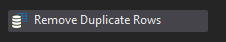# How to see which item is different when comparing two datatables

Hi!

I’ve managed to compare two datatables and send out an alert if there are any differences. However, I would like to know how I can specify which item is incorrect.

Thanks!!

@gabimlobo

Welcome to the UIpath Community.

Use nested For Each Row and then compare it with IF condition.

ForEach row in DT1
ForEach row1 in DT2
If row(“ColumnName”).Tostring = row1(“ColumnName”).Tostring
Then: item is equal
Else: item is not equal

Hello! Thanks for the quick response!!

Could you explain a bit better? I should do that for every column?

1 Like

@gabimlobo

Same you have to write for multiple columns.

IF row(“ColumnName1”).Tostring = row1(“ColumnName1”).Tostring AND row(“ColumnName2”).Tostring = row1(“ColumnName2”).Tostring AND row(“ColumnName3”).Tostring = row1(“ColumnName3”).Tostring

Greetings @gabimlobo,

When you want to find the different rows from each I would do the following:

1. Remove the duplicate rows from each DataTable with2. Merge the 2 DataTables into one with the ‘Merge Data Table’ activity3. In an ‘Assign’ activity write the following sentence in the right:

``````DT.AsEnumerable.GroupBy(Function(row) String.Join(",", row.ItemArray)).Where(Function(g) g.Count < 2).Select(Function(g) g.First).CopyToDataTable
``````

DT is the variable that contains the merged DataTable

On the left part of the ‘Assign’ activity can use any DataTable to assign the result.

The benefit of this, is that you get the difference in one DataTable with just 2 steps.

The following is to explain what is happening in each part of the sentence shown in the second step:

``````DT.AsEnumerable
``````

You are transforming the DataTable to an Enumerable, which is a generic type of collection which is a lot more flexible than DataTables.

``````GroupBy(Function(row) String.Join(",", row.ItemArray))
``````

With this sentence you are transforming every row of the table to an object array (Object[.]), while String.Join will Join all the objects in an array separated with the string given as the first parameter, in this case “,”. Lastly, GroupBy will group all the elements that are the same, in this case because String.Join(“,”, row.ItemArray) this means it will group all rows that are the same.

``````Where(Function(g) g.Count < 2)
``````

Where is a function used to filter on a condition. In here we are filtering out all the groups whose count, or in other words, that contains, 2 or more rows, meaning that these rows were in both of the original DataTables, and therefore are correct. If only one row is found, it won´t be filtered out.

``````Select(Function(g) g.First)
``````

Finally, we want to keep get the rows that were not in both tables, so we pick all the first rows of the groups that weren’t filtered out.

I know it may be a bit complicated, but understanding LINQ will help you a lot if you are working with DataTables in UiPath.

2 Likes

Hi Buddy @gabimlobo

Welcome to uipath community
Fine
–Yes, while comparing either with IF condition or LOOKUP datatable activity whatever along your process, say if you have used excel application scope, read range activity, for each row loop and inside this for each row loop, a IF Condition with some condition in it that validates whether they are equal are not, and if its equal it will go to THEN part of if condition or will go to the ELSE part of if condition
–here inside this ELSE or THEN part (based on the condition that gets passed ) we can have a variable that can store the value of that changing item which can be later used while sending an alert…
–or we can have a column called MATCHES in any one of the excel file, or a source file that is actually compared with, where we can make a update like YES or NO, that depicts that it got matched or not
for that kindly follow the below steps
–use excel application scope and pass the excel file path of first file as input
–use a read range activity within this scope and get the output with a variable of type datatable named outdt1
–use another excel application scope and pass the file path of second file as input
–use again a read range activity within this excel application scope and get the output as outdt2
–next to this second excel application scope use a for each row loop and pass the input outd1
–within this loop use another for each row loop and pass the input as outdt2
–now inside this inner for each row loop use a if condition put all your condition and validate which will take to either THEN or ELSE
–say it goes to ELSE part if there is a mismatch FOUND, so here inside the ELSE part, use a write cell activity and if we want to update the column E (for example), then in the range mention as “E”+Counter.ToString and in the value mention as “DOESN’T MATCHES”
–Counter is the variable of type int32, with the default value 2 defined in the variable panel
–Next to this WRITE CELL activity we need to use a assign activity outside this inner for each row loop (the sequence should be like this, first a for each row loop, within this secondly another for each row loop, use a if condition inside second for each row loop, then write cell workbook activity mentioning the cell range and the workbook as EXCEL1 (first excel - the source file) not excel2, after which use a assign activity outside the second the for each row loop ) to increment the value of counter to make sure that we type into the next cell, next row in the column for that we need to increment the value
counter = counter + 1

Thats all buddy